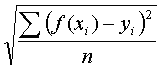# Root Mean Squared Error

If you have some data and try to make a curve (a formula) fit them, you can graph and see how close the curve is to the points. Another measure of how well the curve fits the data is Root Mean Squared Error.

For each data point, CalGraph calculates the value of  y from the formula. It subtracts this from the data's y-value and squares the difference. All these squares are added up and the sum is divided by the number of data. Finally CalGraph takes the square root. Written mathematically, Root Mean Square Error isRoot Mean Squared Error is calculated automatically when you click Calculate on either Exponential Curve Fitting or Polynomial Curve Fitting.